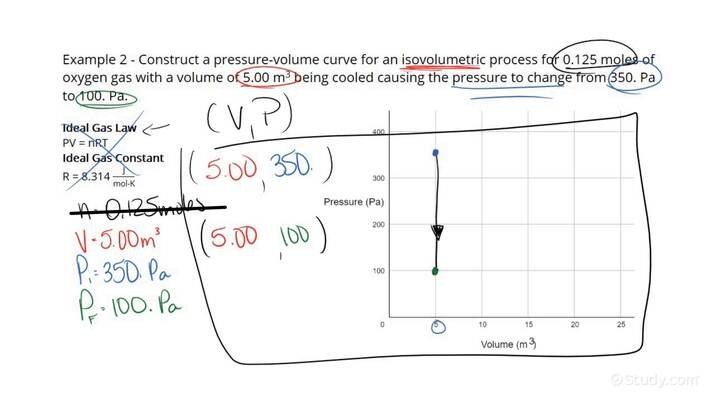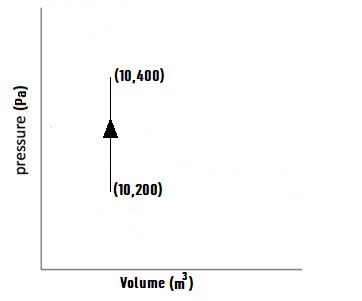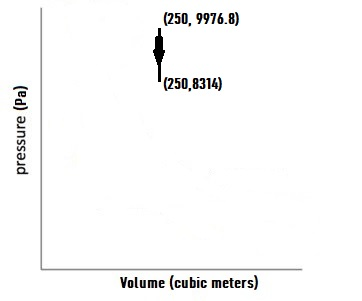# How to Draw an Isovolumetric Process on a Pressure-Volume DiagramAn error occurred trying to load this video.

Try refreshing the page, or contact customer support.

• 0:12 Example 1
• 4:18 Example 2
Rishabh Singh, Kirsten Wordeman
• Instructors
Rishabh Singh

Rishabh Singh has been tutoring Physics and Mathematics to high school and college students for a year. Rishabh completed a Bachelor of Science in Physics and Applied Mathematics with honors from UCLA.

•
Kirsten Wordeman

Kirsten has taught high school biology, chemistry, physics, and genetics/biotechnology for three years. She has a Bachelor's in Biochemistry from The University of Mount Union and a Master's in Biochemistry from The Ohio State University. She holds teaching certificates in biology and chemistry.

## How to Draw an Isovolumetric Process on a Pressure-Volume Diagram

Step 1: Determine the volume at which the process is occurring. Also determine the initial and final pressures of the gas after the process. If this is not provided directly, and instead the initial and final temperatures of the gas, along with the number of moles of gas have been provided, use the ideal gas law to determine the initial and final pressures, as {eq}PV = nRT \text{ ( the ideal gas law) }\\ \implies P = \frac{nRT}{V} \\ \implies P_{1} = \frac{nRT_{1}}{V} \text{ and } P_{2} = \frac{nRT_{2}}{V} {/eq}, where P is the pressure on the gas, n is the number of moles of gas, R is the ideal gas constant, and T is the temperature of the gas. The subscripts 1 and 2 are used to denote the initial and final states of the gas respectively.

Step 2: To draw the isovolumetric process on the pressure-volume diagram, simply draw a straight vertical line at an x value of x = V, and extending from the initial pressure {eq}P_{1} {/eq} to final pressure {eq}P_{2} {/eq}, and pointing towards the final pressure.

## What are Isovolumetric Processes, Pressure-Volume diagrams and the Ideal gas law?

Isovolumetric processes: Isovolumetric processes are thermodynamic in which the volume of the system (i.e. the volume occupied by the ideal gas) does not change.

Pressure-Volume Diagrams: Pressure-Volume Diagrams are graphs that have pressure exerted by the gas as the y-axis, and the volume occupied by the gas as the x-axis. These diagrams are used to represent thermodynamic processes that effect change in the state of the gas.

Ideal gas law: The ideal gas law simply related the pressure, volume, number of moles, and temperature of an ideal gas. Mathematically, it takes the form {eq}PV = nRT \text{ ( the ideal gas law) }\\ \implies P = \frac{nRT}{V} {/eq},where P is the pressure on the gas, n is the number of moles of gas, R is the ideal gas constant whose value is 8.314 {eq}J\cdot mol^{-1} \cdot K^{-1} {/eq}, and T is the temperature of the gas.

## Example Problems:

Example 1 Drawing an isovolumetric process given the pressure values directly

Q: Draw a pressure-volume curve for an isovolumetric process of oxygen in a rigid container with volume 10 cubic meters being heated up, if the reading of a barometer within the container goes from 200 Pa to 400 Pa.

Step 1: We determine that the volume at which the process is occurring is 10 {eq}m^{3} {/eq}. Also we determine the initial and final pressures of the gas after the process to be {eq}P_{1} = 200 Pa \text{ and } P_{2} = 400 Pa {/eq} respectively.

Step 2: To draw the isovolumetric process on the pressure-volume diagram, simply draw a straight vertical line at an x value of x = 10, extending from the initial pressure {eq}P_{1} = 200 {/eq} to final pressure {eq}P_{2} = 400 {/eq}, and pointing towards the final pressure, as shown below:Example 2 Drawing an isovolumetric process given the temperature values

Q: Draw a pressure-volume curve for an isovolumetric process of 2 moles of chlorine gas in a rigid container with volume 250 cubic meter being cooled, if the reading of a thermometer within the container goes from 600 K to 500 K.

Step 1: We know the volume at which the process is occurring is 1 {eq}m^{3} {/eq}. Now we determine the initial and final pressures of the gas after the process. As this is not provided directly, and instead the initial and final temperatures of the gas, along with the number of moles of gas have been provided, we use the ideal gas law to determine the initial and final pressures, as {eq}PV = nRT \text{ ( the ideal gas law) }\\ \implies P = \frac{nRT}{V} \\ \implies P_{1} = \frac{nRT_{1}}{V} \text{ and } P_{2} = \frac{nRT_{2}}{V} \\ \implies P_{1} = \frac{2\times 8.314\times 600}{1} \text{ and } P_{2} = \frac{2\times 8.314\times 500}{1}\\ \implies P_{1} = 9976.8 Pa \text{ and } P_{2} = 8314 Pa {/eq}, where P is the pressure on the gas, n is the number of moles of gas, R is the ideal gas constant, and T is the temperature of the gas. The subscripts 1 and 2 are used to denote the initial and final states of the gas respectively.

Step 2: To draw the isovolumetric process on the pressure-volume diagram, simply draw a straight vertical line at an x value of x = 1, and extending from the initial pressure {eq}P_{1} = 9976.8 Pa {/eq} to final pressure {eq}P_{2} = 8314 Pa {/eq}, and pointing towards the final pressure, as :